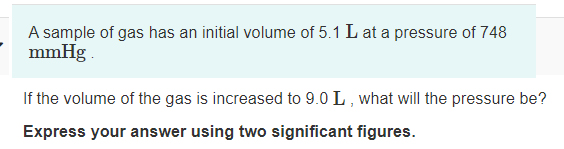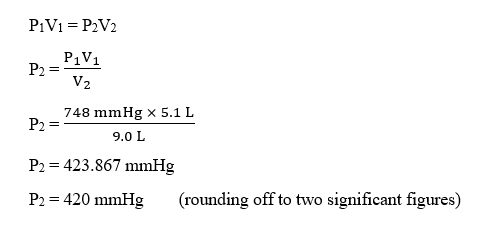# A sample of gas has an initial volume of 5.1 L at a pressure of 748mmHgIf the volume of the gas is increased to 9.0 L , what will the pressure be?Express your answer using two significant figures.

Question
58 viewshelp_outlineImage TranscriptioncloseA sample of gas has an initial volume of 5.1 L at a pressure of 748 mmHg If the volume of the gas is increased to 9.0 L , what will the pressure be? Express your answer using two significant figures. fullscreen
check_circle

Step 1

Given,

Initial volume, V1 = 5.1 L

Initial pressure, P1 = 748 mmHg

Final volume, V2 = 9.0 L

Final pressure, P2 = ?

Step 2

The final pressure can be calculated using Boyle’s law :...

### Want to see the full answer?

See Solution

#### Want to see this answer and more?

Solutions are written by subject experts who are available 24/7. Questions are typically answered within 1 hour.*

See Solution
*Response times may vary by subject and question.
Tagged in